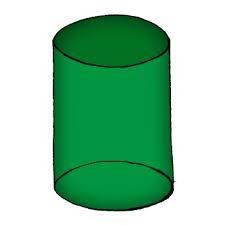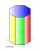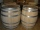# Container

The container has a cylindrical shape the base diameter 0.8 m and the area of the base is equal to the area of the wall. How many liters of water can we pour into the container?

Correct result:

V =  100.531 l

#### Solution:We would be pleased if you find an error in the word problem, spelling mistakes, or inaccuracies and send it to us. Thank you!Tips to related online calculators
Do you know the volume and unit volume, and want to convert volume units?

## Next similar math problems:

• VintnerHow high can vintner fill keg with crushed red grapes if these grapes occupy a volume of 20 percent? Keg is cylindrical with a diameter of the base 1 m and a volume 9.42 hl. Start from the premise that says that fermentation will fill the keg (the number
• Cylinder containerThe cylindrical container with a diameter of 1.8 m contains 2,000 liters of water. How high does the water reach?
• Cylindrical tank9.6 hl of water is poured into a cylindrical tank with a bottom diameter of 1.2 m. What height in centimeters does the water reach?
• The cylindrical containerThe container has a cylindrical shape the base diameter 0.8 meters has a content area of the base is equal to the content area of the shell. How many full liters of water can be poured maximally into the container?
• Cylindrical tankCylindrical tank holds 600hl water and is deep 2.5 m. Calculate the diameter of the cylinder.
• The coilHow many ropes (the diameter 8 mm) fit on the coil (threads are wrapped close together) The coil has dimension: the inner diameter 400mm, the outside diameter 800mm and the length of the coil is 470mm
• Height as diameter of baseThe rotary cylinder has a height equal to the base diameter and the surface of 471 cm2. Calculate the volume of a cylinder.
• Octagonal prism vase0.7 l of water can be poured in an octagonal prism vase. What is the height of the vase, if the bottom has a area of 25 cm square and a thickness of 12 mm?
• Water in aquariumThe aquarium cuboid shape with a length of 25 cm and a width of 30 cm is 9 liters of water. Calculate the areas which are wetted with water.
• Fuel economyHow many kilometers is sufficient petrol in the cylinder fuel tank with a diameter 40 cm and the base of tank length 1 m, when it is filled to 60% and if the car consume 15 liters per 100 km?
• Tank diameterA cylindrical tank has a volume of 60 hectoliters and is 2.5 meters deep. Calculate the tank diameter.
• 3d printer3D printing ABS filament with diameter 1.75 mm has density 1.04 g/cm3. Find the length of m = 5 kg spool filament. (how to calculate length)
• PebbleThe aquarium with internal dimensions of the bottom 40 cm × 35 cm and a height of 30 cm is filled with two-thirds of water. Calculate how many millimeters the water level in the aquarium rises by dipping a pebble-shaped sphere with a diameter of 18 cm.
• Four prismsQuestion No. 1: The prism has the dimensions a = 2.5 cm, b = 100 mm, c = 12 cm. What is its volume? a) 3000 cm2 b) 300 cm2 c) 3000 cm3 d) 300 cm3 Question No.2: The base of the prism is a rhombus with a side length of 30 cm and a height of 27 cm. The heig
• Ratio of volumesIf the heights of two cylindrical drums are in the ratio 7:8 and their base radii are in the ratio 4:3. What is the ratio of their volumes?
• Two cylindersTwo cylinders are there one with oil and one with an empty oil cylinder has no fixed value assume infinitely. We are pumping out the oil into an empty cylinder having radius =1 cm height=3 cm rate of pumping oil is 9 cubic centimeters per sec and we are p
• TentCalculate how many liters of air will fit in the tent that has a shield in the shape of an isosceles right triangle with legs r = 3 m long the height = 1.5 m and a side length d = 5 m.